CLO Standalone 4.0.131 WIN三维可视化服装设计软件 中文注册版

CLO Standalone 4.0.131 WIN三维可视化服装设计软件 中文注册版

CLO Standalone 4.0.131 是一款专门用来作为时尚产品的可视化工具，CLO拥有强大的模拟技术和10多年来尖端计算机图形技术研发的成果，使您能够创建多层和复杂细节的服装，从简单的上衣到含有复杂配饰和结构的外套，CLO还可以生成帽子，箱包，钱包，内衣，泳衣等一切由面料制成的东西，CLO复杂算法可以模拟织物的物理性质，以精确地想象它们在现实生活中的样子。This is 100% 3D. And the result of over 10 years of R&D in cutting-edge computer graphics technology. Our sophisticated algorithm simulates the physical properties of fabrics to accurately visualize the way they drape in real-life.

主要功能：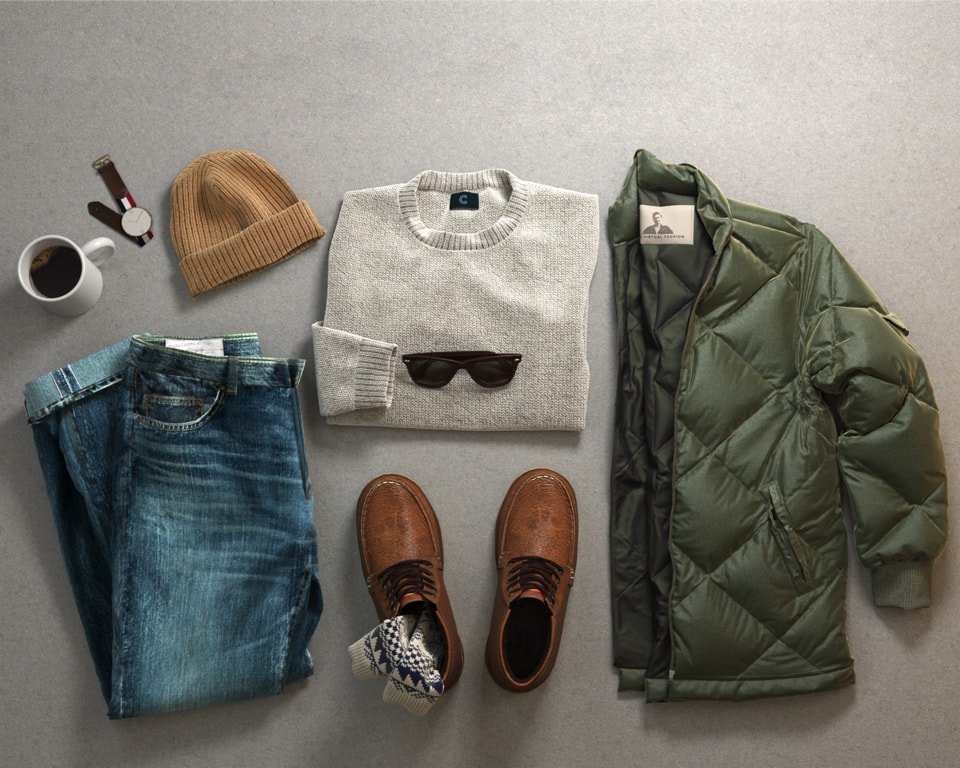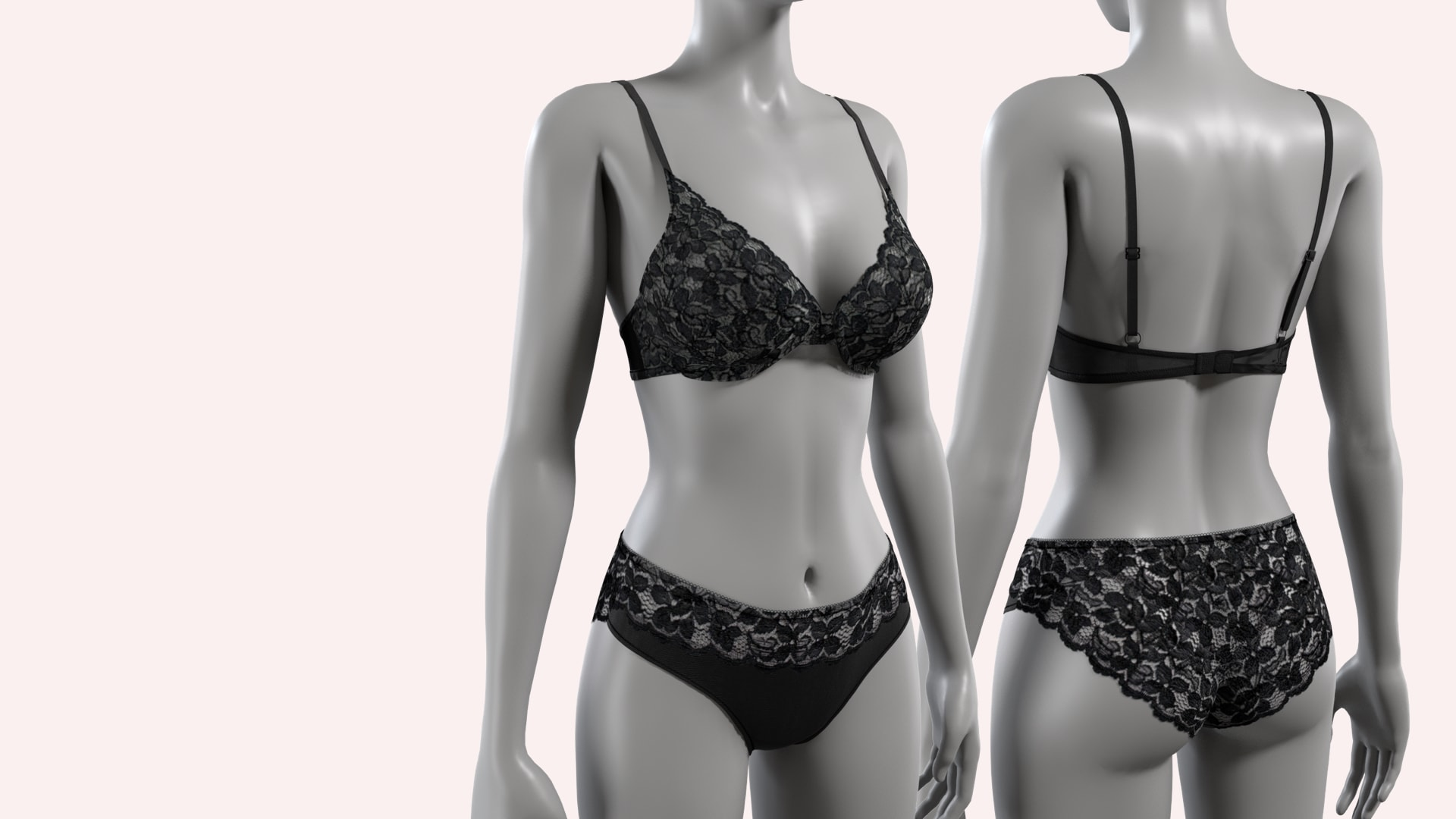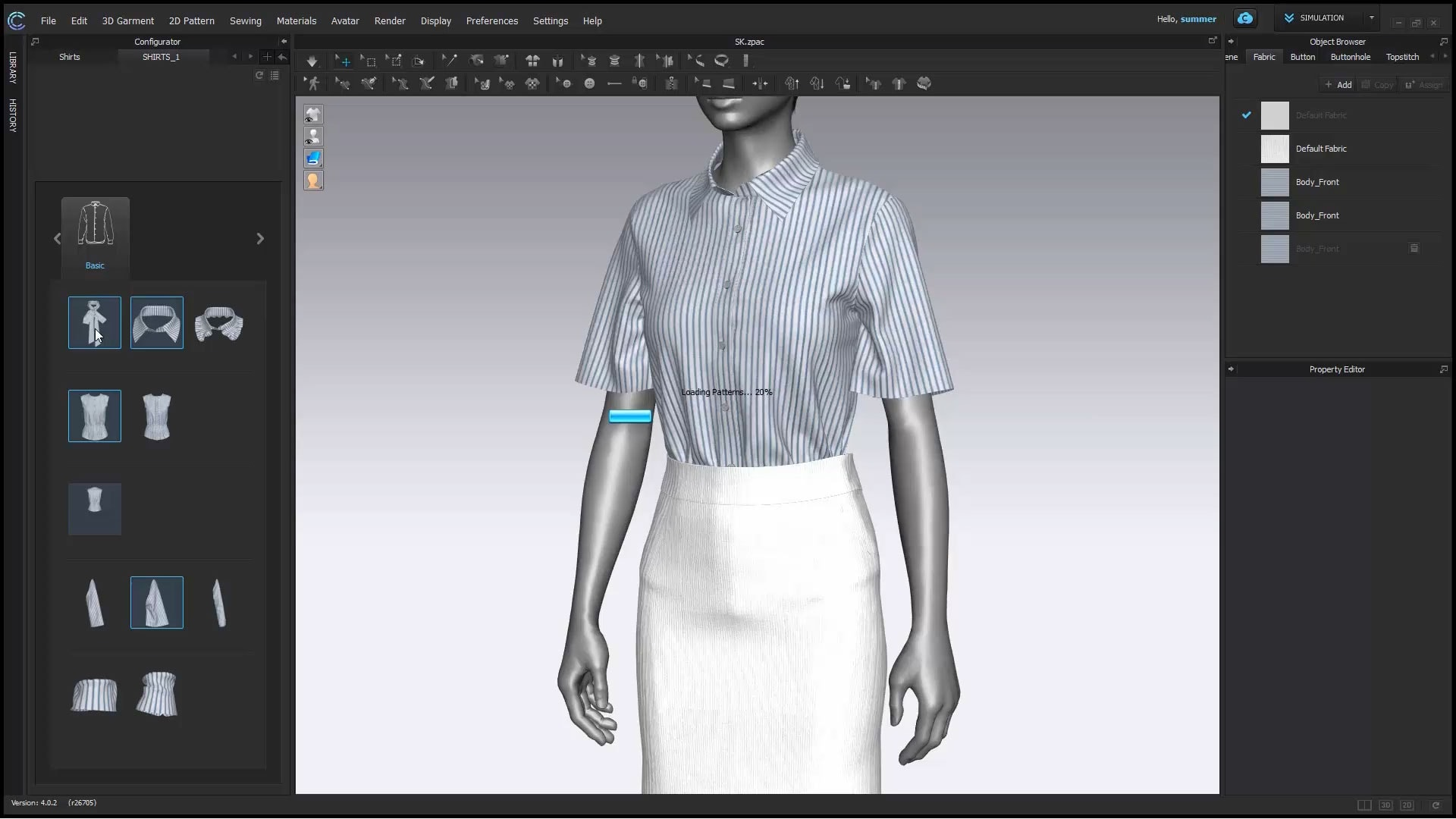CLO可以准确模拟面料的悬垂性，例如具有不同物理属性的轻质平纹和针织面料。访问CLO全面的常用织物库，立即看到效果。可根据需求应用粘衬、粘衬条和归拔等技术，以调整3D服装的合身性。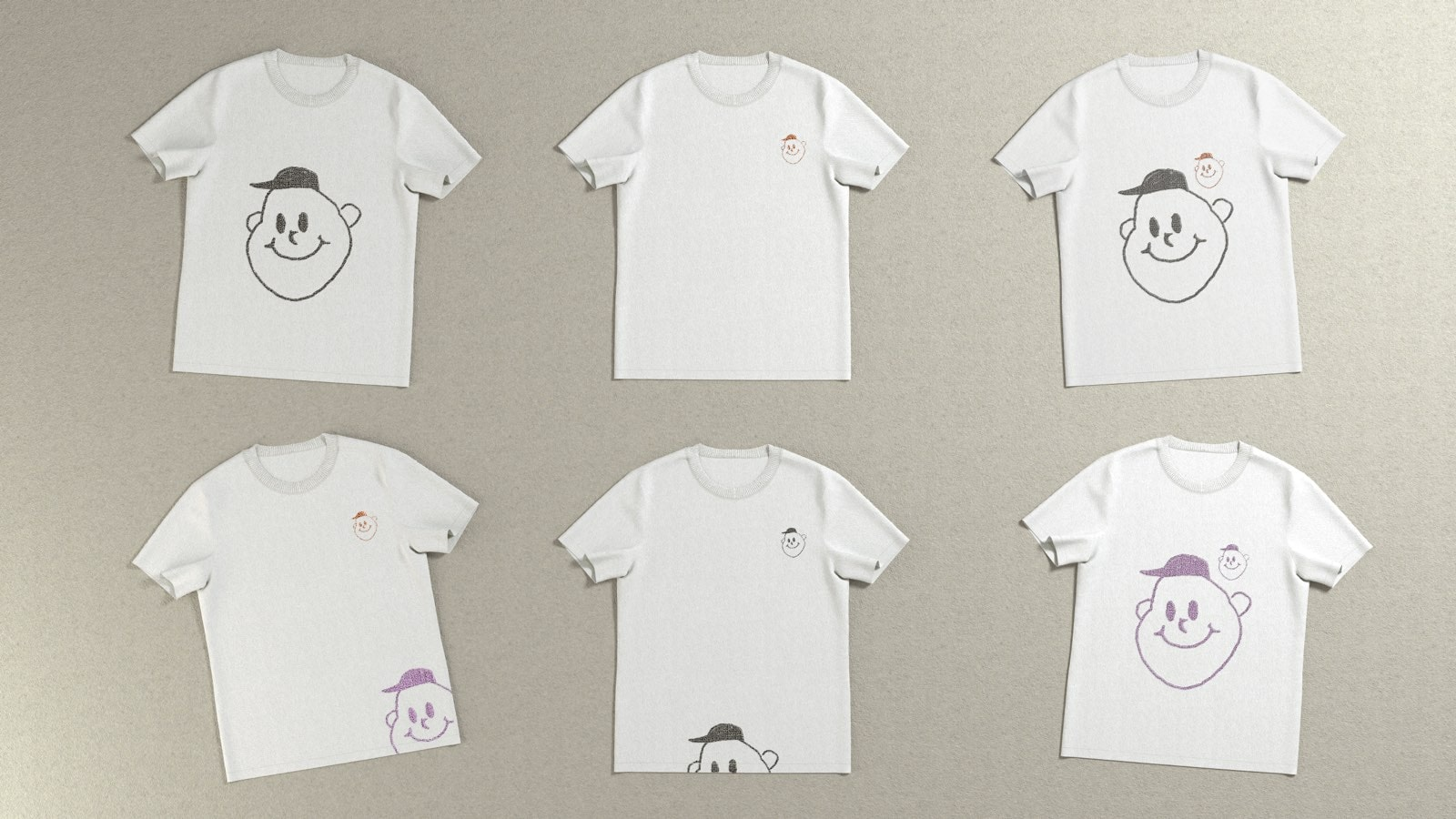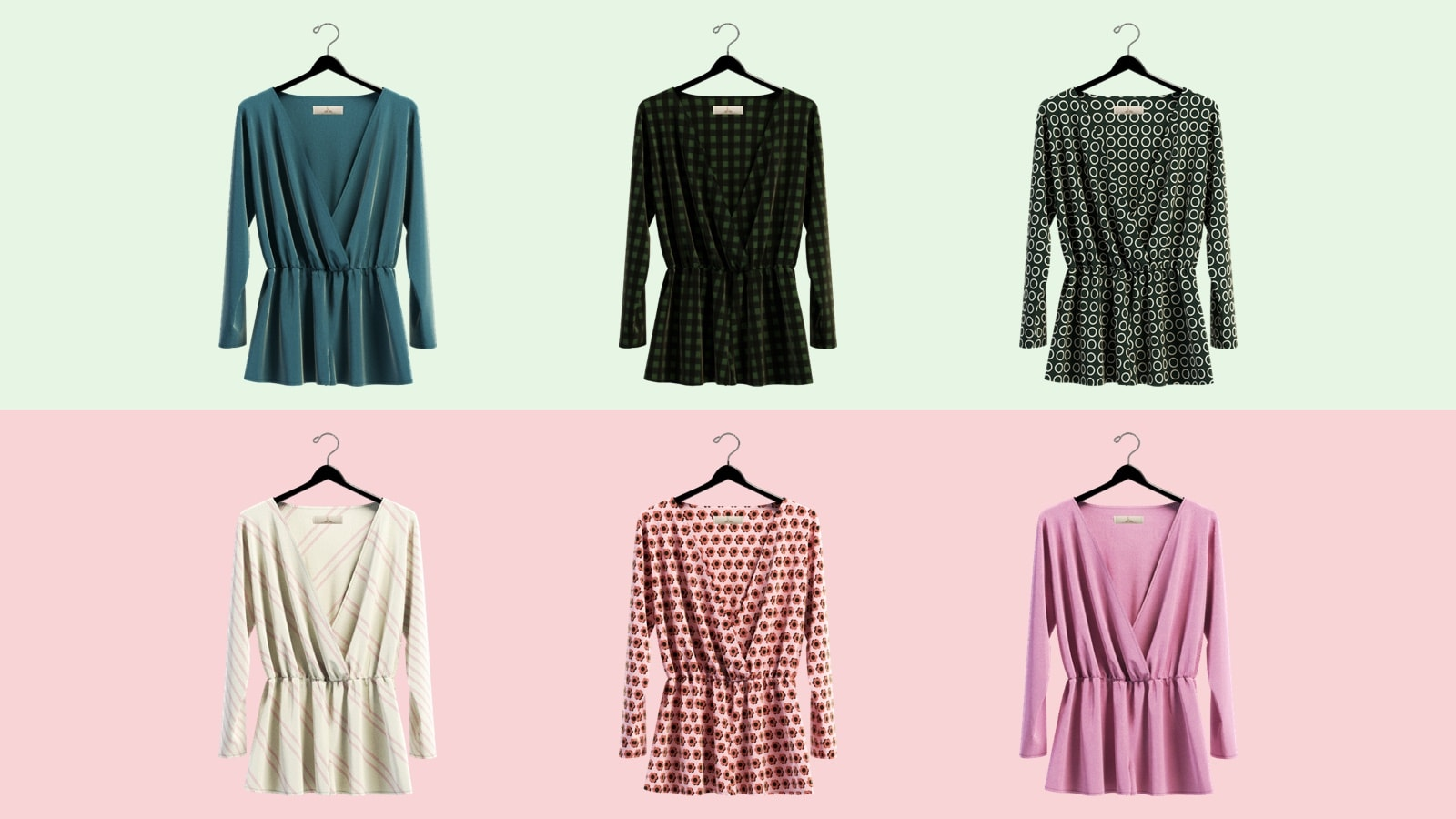安装方法：1.安装软件CLO_Standalone_OnlineAuth_4_0_131_Installer_x64.exe2.复制CLO_Standalone_OnlineAuth_x64.exe和SA.reg文件到软件安装目录下3.运行SA.reg注册表，即可，使用防火墙工具软件Fab，屏蔽软件联网Block4.51render恭喜你，安装成功，祝你使用愉快

评论

• 😀
• 😬
• 😁
• 😂
• 😃
• 😄
• 😅
• 😆
• 😇
• 😉
• 😊
• 😋
• 😌
• 😍
• 😘
• 😗
• 😙
• 😚
• 😜
• 😝
• 😛
• 😎
• 😏
• 😶
• 😐
• 😑
• 😒
• 😳
• 😞
• 😟
• 😠
• 😡
• 😔
• 😕
• 😣
• 😖
• 😫
• 😩
• 😤
• 😮
• 😱
• 😨
• 😰
• 😯
• 😦
• 😧
• 😢
• 😥
• 😪
• 😓
• 😭
• 😵
• 😲
• 😷
• 😴
• 💤
• 💩
• 😈
• 👿
• 👹
• 👺
• 💀
• 👻
• 👽
• 😺
• 😸
• 😹
• 😻
• 😼
• 😽
• 🙀
• 😿
• 😾
• 🙌
• 👏
• 👋
• 👍
• 👎
• 👊
• 👌
• 👐
• 💪
• 🙏
• 👇
• 👈
• 👉
• 🖖
• 💅
• 👄
• 👅
• 👂
• 👃
• 👀
• 👤
• 👥
• 👶
• 👦
• 👧
• 👨
• 👩
• 👱
• 👴
• 👲
• 👳
• 👮
• 👷
• 💂
• 🎅
• 👼
• 👸
• 👰
• 🚶
• 🏃
• 💃
• 👯
• 👫
• 👬
• 👭
• 🙇
• 💁
• 🙅
• 🙆
• 🙋
• 🙎
• 🙍
• 💇
• 💆
• 💑
• 👩
• 👩
• 👨
• 👨
• 💏
• 👩
• 💋
• 👩
• 👨
• 💋
• 👨
• 👪
• 👨
• 👚
• 👕
• 👖
• 👔
• 👗
• 👙
• 👘
• 💄
• 💋
• 👣
• 👠
• 👡
• 👢
• 👞
• 👟
• 👒
• 🎩
• 🎓
• 👑
• 🎒
• 👝
• 👛
• 👜
• 💼
• 👓
• 💍
• 🌂
• 🐶
• 🐱
• 🐭
• 🐹
• 🐰
• 🐻
• 🐼
• 🐨
• 🐯
• 🐮
• 🐷
• 🐸
• 🐙
• 🐵
• 🙈
• 🙉
• 🙊
• 🐒
• 🐔
• 🐧
• 🐦
• 🐤
• 🐣
• 🐥
• 🐺
• 🐗
• 🐴
• 🐝
• 🐛
• 🐌
• 🐞
• 🐜
• 🐍
• 🐢
• 🐠
• 🐟
• 🐡
• 🐬
• 🐳
• 🐋
• 🐊
• 🐆
• 🐅
• 🐃
• 🐂
• 🐄
• 🐪
• 🐘
• 🐐
• 🐏
• 🐑
• 🐎
• 🐖
• 🐀
• 🐁
• 🐓
• 🐩
• 🐈
• 🐇
• 🐾
• 🐉
• 🐲
• 🌵
• 🌲
• 🌳
• 🌴
• 🌱
• 🌿
• 🍀
• 🎍
• 🎋
• 🍃
• 🍂
• 🍁
• 🌾
• 🌺
• 🌻
• 🌹
• 🌷
• 🌼
• 🌸
• 💐
• 🍄
• 🌰
• 🎃
• 🐚
• 🌎
• 🌍
• 🌏
• 🌕
• 🌖
• 🌘
• 🌑
• 🌒
• 🌓
• 🌔
• 🌚
• 🌝
• 🌛
• 🌜
• 🌞
• 🌙
• 🌟
• 💫
• 🔥
• 💥
• 💧
• 💦
• 🌊
• 🍏
• 🍎
• 🍐
• 🍊
• 🍋
• 🍌
• 🍉
• 🍇
• 🍓
• 🍈
• 🍒
• 🍑
• 🍍
• 🍅
• 🍆
• 🌽
• 🍠
• 🍯
• 🍞
• 🍗
• 🍖
• 🍤
• 🍳
• 🍔
• 🍟
• 🍕
• 🍝
• 🍜
• 🍲
• 🍥
• 🍣
• 🍱
• 🍛
• 🍙
• 🍚
• 🍘
• 🍢
• 🍡
• 🍧
• 🍨
• 🍦
• 🍰
• 🎂
• 🍮
• 🍬
• 🍭
• 🍫
• 🍩
• 🍪
• 🍺
• 🍻
• 🍷
• 🍸
• 🍹
• 🍶
• 🍵
• 🍼
• 🍴
• 🏀
• 🏈
• 🎾
• 🏉
• 🎱
• 🎿
• 🏂
• 🎣
• 🚣
• 🏊
• 🏄
• 🛀
• 🚴
• 🚵
• 🏇
• 🏆
• 🎽
• 🎫
• 🎭
• 🎪
• 🎤
• 🎧
• 🎼
• 🎹
• 🎷
• 🎺
• 🎸
• 🎻
• 🎬
• 🎮
• 👾
• 🎯
• 🎲
• 🎰
• 🎳
• 🚗
• 🚙
• 🚌
• 🚎
• 🚓
• 🚑
• 🚒
• 🚐
• 🚚
• 🚛
• 🚜
• 🚲
• 🚨
• 🚔
• 🚍
• 🚘
• 🚖
• 🚡
• 🚠
• 🚟
• 🚃
• 🚋
• 🚝
• 🚄
• 🚅
• 🚈
• 🚞
• 🚂
• 🚆
• 🚇
• 🚊
• 🚉
• 🚁
• 🚤
• 🚀
• 💺
• 🚧
• 🚏
• 🚦
• 🚥
• 🚢
• 🎡
• 🎢
• 🎠
• 🌁
• 🗼
• 🏭
• 🎑
• 🗻
• 🌋
• 🗾
• 🌅
• 🌄
• 🌇
• 🌆
• 🌃
• 🌉
• 🌌
• 🌠
• 🎇
• 🎆
• 🌈
• 🏰
• 🏯
• 🗽
• 🏠
• 🏡
• 🏢
• 🏬
• 🏣
• 🏤
• 🏥
• 🏨
• 🏪
• 🏫
• 🏩
• 💒
• 💛
• 💚
• 💙
• 💜
• 💔
• 💕
• 💞
• 💓
• 💗
• 💖
• 💘
• 💝
• 💟
• 🔯
• 🆔
• 🈳
• 🈹
• 📴
• 📳
• 🈶
• 🈚
• 🈸
• 🈺
• 🆚
• 🉑
• 💮
• 🉐
• 🈴
• 🈵
• 🈲
• 🆑
• 🆘
• 📛
• 🚫
• 💢
• 🚷
• 🚯
• 🚳
• 🚱
• 🔞
• 📵
• 💯
• 🔅
• 🔆
• 🔱
• 🚸
• 🔰
• 🈯
• 💠
• 🌀
• 🌐
• 🏧
• 🈂
• 🛃
• 🛄
• 🚭
• 🚾
• 🚰
• 🚹
• 🚺
• 🚼
• 🚻
• 🚮
• 🎦
• 📶
• 🈁
• 🆖
• 🆗
• 🆙
• 🆒
• 🈁
• 🆓
• 🔢
• 🔀
• 🔁
• 🔂
• 🔼
• 🔽
• 🔄
• 🔤
• 🔡
• 🔠
• 🔣
• 🔣
• 🎵
• 🎵
• 🎶
• 🔃1714 | 0